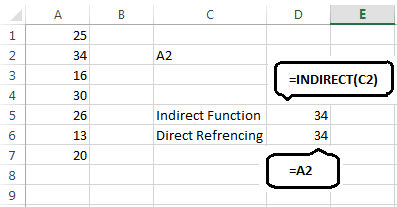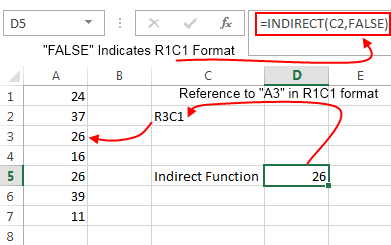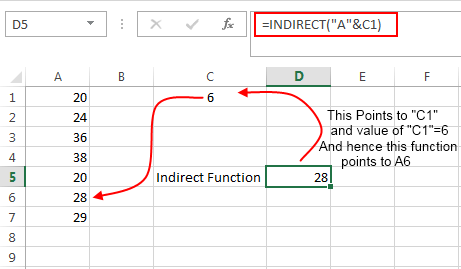# Excel Indirect Function – How to Use

Excel Indirect Function is one of the most useful functions in Microsoft Excel. Indirect function takes a cell reference in the form of a string, then it evaluates the reference and shows its content. This gives you a better way to deal with variable cell references.

### Excel Indirect Function Syntax:

The syntax of indirect function is quite simple:

`INDIRECT(ref_text,a1)`

Here ‘ref_text’ specifies a string that points to a cell reference.

‘a1’ specifies a Boolean value i.e. TRUE or FALSE

• If the value is FALSE this means that the cell references are in R1C1 format.
• If it is TRUE this means that the cell references are in A1-style.

If the second parameter is left blank then it is treated to be true by default.

### Example of Indirect Function:

An example of Indirect Function in shown in the below image.Here an indirect function is written that points to cell “C2”.

The “C2” cell in turn points to “A6” and the value of “A6” cell is 27. And hence the indirect function used in the example results into 27.

### Why to Choose Indirect Function over Direct Referencing:

If you don’t know about direct referencing in excel then I must tell you that it is a method that looks very similar to Indirect function. To perform direct referencing simple enter a cell reference prefixed by an “=” sign. E.g. `‘=A6’`

As we all know that indirect referencing can help to deal you with variable cell reference in a better way. This is not true in the case of direct referencing.In the above example if you have a table as shown in the above image and you have a direct reference to the cell “A2” and an Indirect reference by using the Indirect function. Now at this instance both these fetch you the same result.

But if you inset a new row at A2 position, then the direct reference to A2 will now point to cell “A3” whereas the indirect reference does not change. This is called locking of a reference.

### Using Excel Indirect formula for R1C1 References:

Using Excel Indirect formula for locating R1C1 references is easy. R1C1 is a format where both the rows and columns are defined using numbers. R stands for Row and C stands for column. R2C3 stands for Row 2 and Column 3 i.e. C2. You can read more about R1C1 references here.In the above image I have used the Indirect Function as:

`=INDIRECT(C2,FALSE)`

In this C2 indicates the reference and the second parameter “FALSE” indicates that the reference is in R1C1 format. On the C2 cell the value is R3C1, which means that it is pointing to Row 3 and column 1. And the value at Row 3 and Column 1 is 26.

### Indirect function using references from two different strings:

You can also supply the references to the indirect function from two separate text strings. For instance you can also write the Indirect function as in the below snapshot.In the above example the Indirect function is written as:

`=INDIRECT("A"&C1)`

This tells Microsoft excel to take half the reference (The alphabet part) as “A” and the other half (the numeric part) from the cell “C1”. In the above example the cell “C1” contains 6 and thus the reference results into “A6” which has a value: 28. And that’s what the indirect function in the example fetches.

This was all about using Excel Indirect function.About Ankit Kaul

Ankit is the founder of Excel Trick. He is tech Geek who loves to sit in front of his square headed girlfriend (his PC) all day long. :D. Ankit has a strong passion for learning Microsoft Excel. His only aim is to turn you guys into 'Excel Geeks'.